Question

A turbo pump transfers water from tank 1 to tank to tank 2 as shown in...

A turbo pump transfers water from tank 1 to tank to tank 2 as shown in the picture below. The diameter of the piping system is D= 4in and the total length of pipes is L=620 ft. the elevation difference is Z1-Z2= 30ft. Use the chart for KL values.

a) determine the flow rate (Q)

b) power of the pump in HP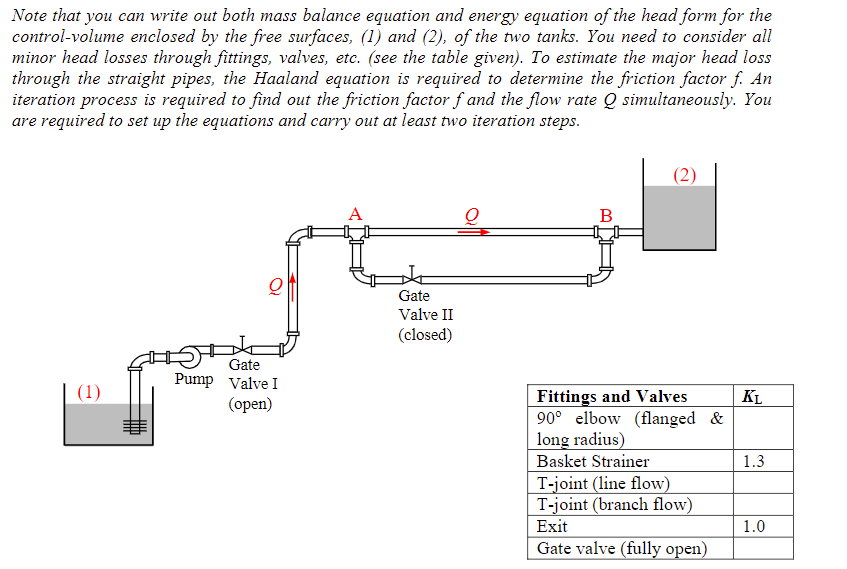Note that you can write out both mass balance equation and energy equation of the head form for the control-volume enclosed by the free surfaces, (1) and (2), of the two tanks. You need to consider all minor head losses through fittings, valves, etc. (see the table given). To estimate the major head loss through the straight pipes, the Haaland equation is required to determine the friction factor f. An iteration process is required to find out the friction factor f and the flow rate O simultaneously. You are required to set up the equations and carry out at least two iteration steps. (2) A B (0 Gate Valve II (closed) (1) Gate Pump Valve I (open) KL 1.3 Fittings and Valves 90° elbow (flanged & long radius) Basket Strainer T-joint (line flow) T-joint (branch flow) Exit Gate valve (fully open) 1.0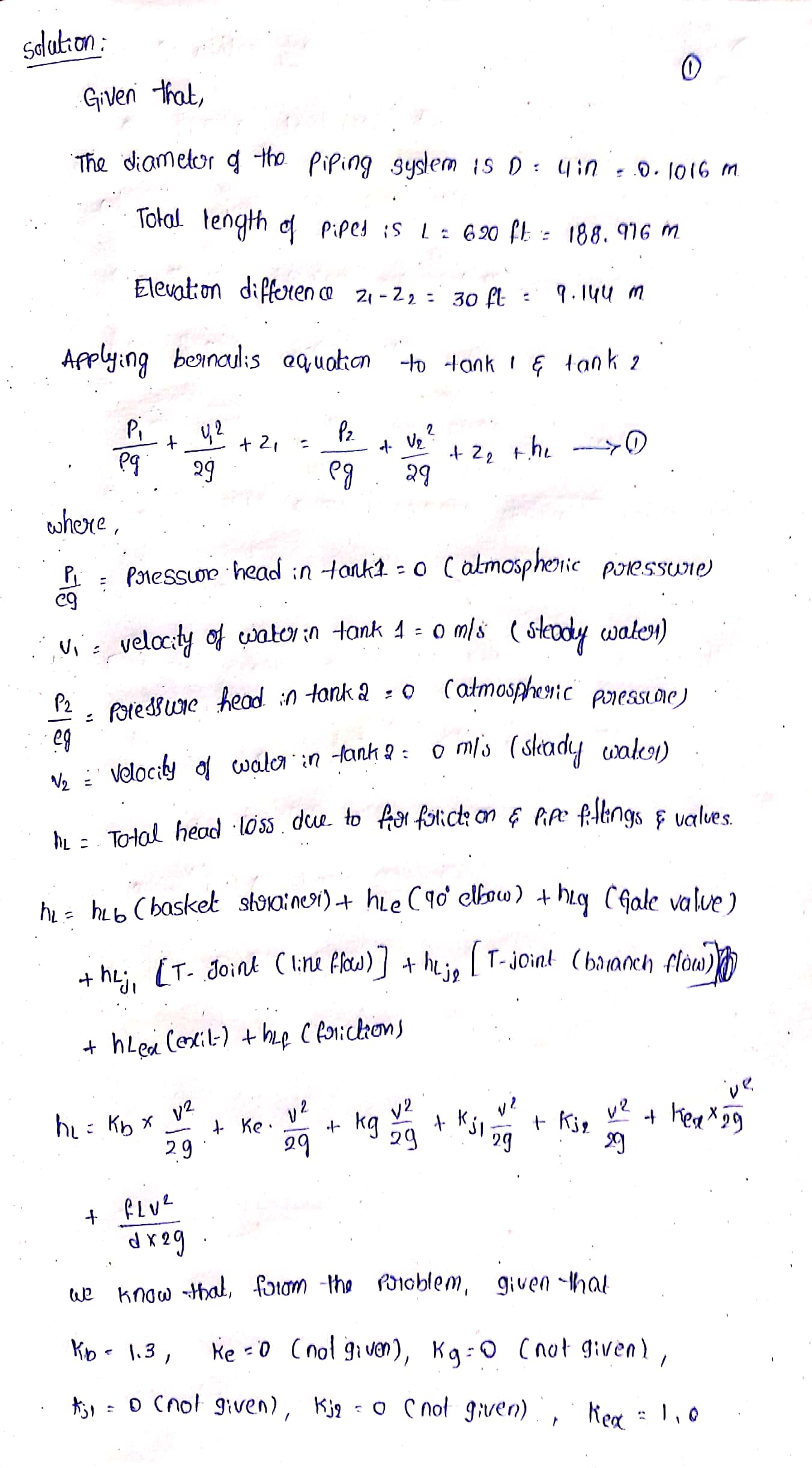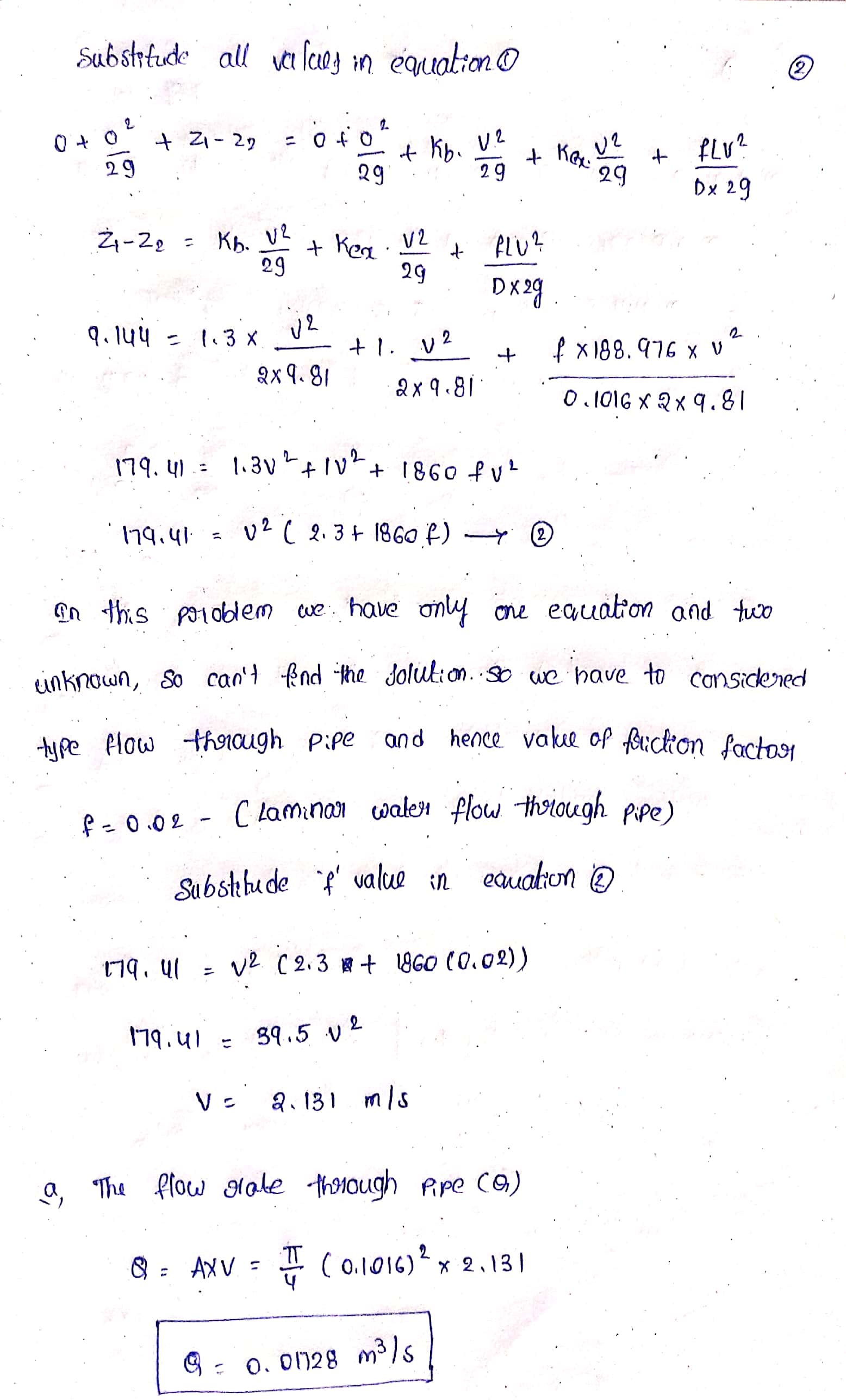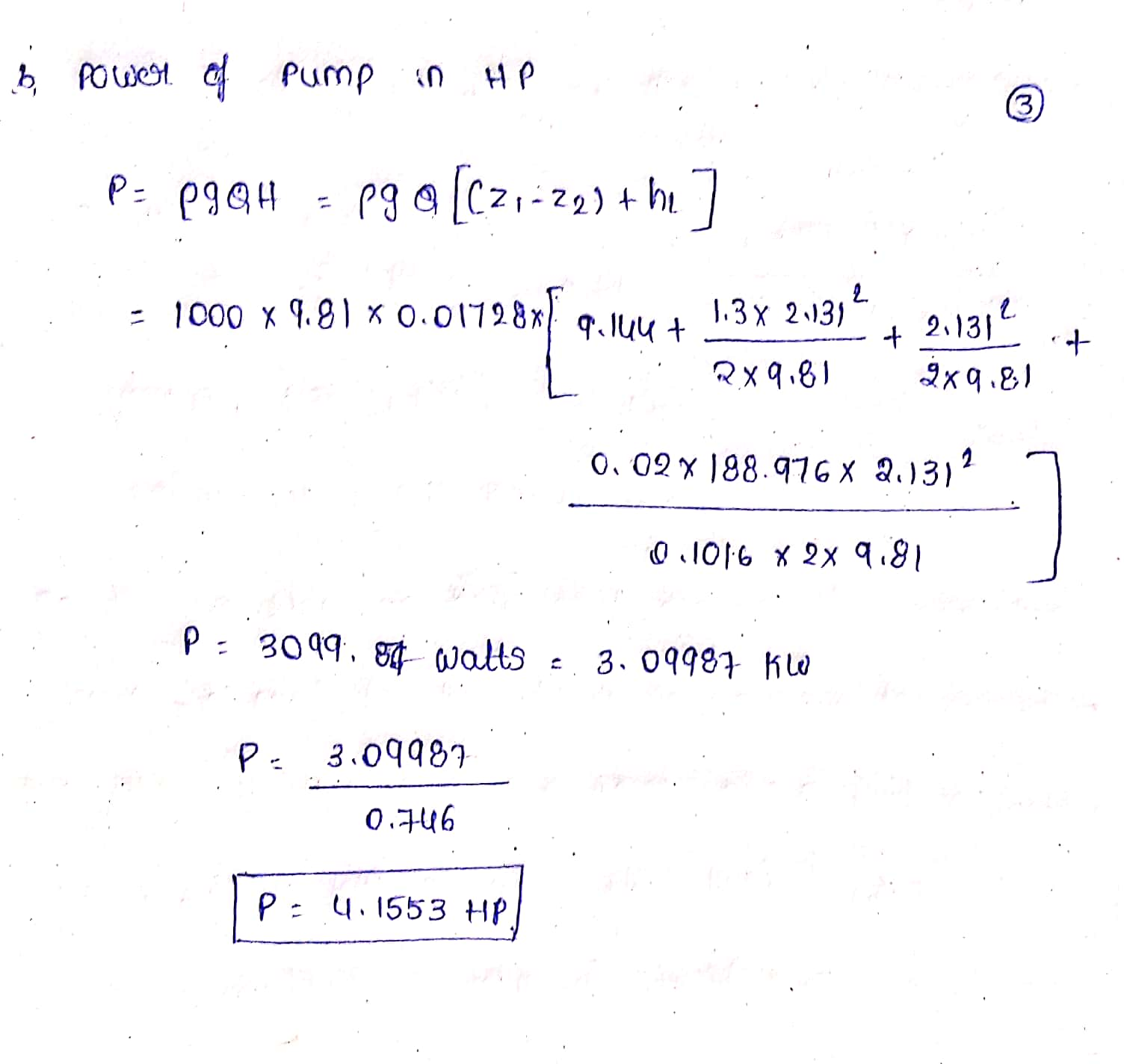please give some positive rating..I have less cf score..thank you so much..

Earn Coins

Coins can be redeemed for fabulous gifts.

Similar Homework Help Questions
• A turbo pump transfers water from tank 1 to tank to tank 2 as shown in...A turbo pump transfers water from tank 1 to tank to tank 2 as shown in the picture below. The diameter of the piping system is D= 4in and the total length of pipes is L=620 ft. the elevation difference is Z1-Z2= 30ft. Use the chart for KL values. a) determine the flow rate (Q) b) power of the pump in HP Note that you can write out both mass balance equation and energy equation of the head form for...

• A pump transports water from Tank 1 to Tank 2 through a constant-diameter piping system as...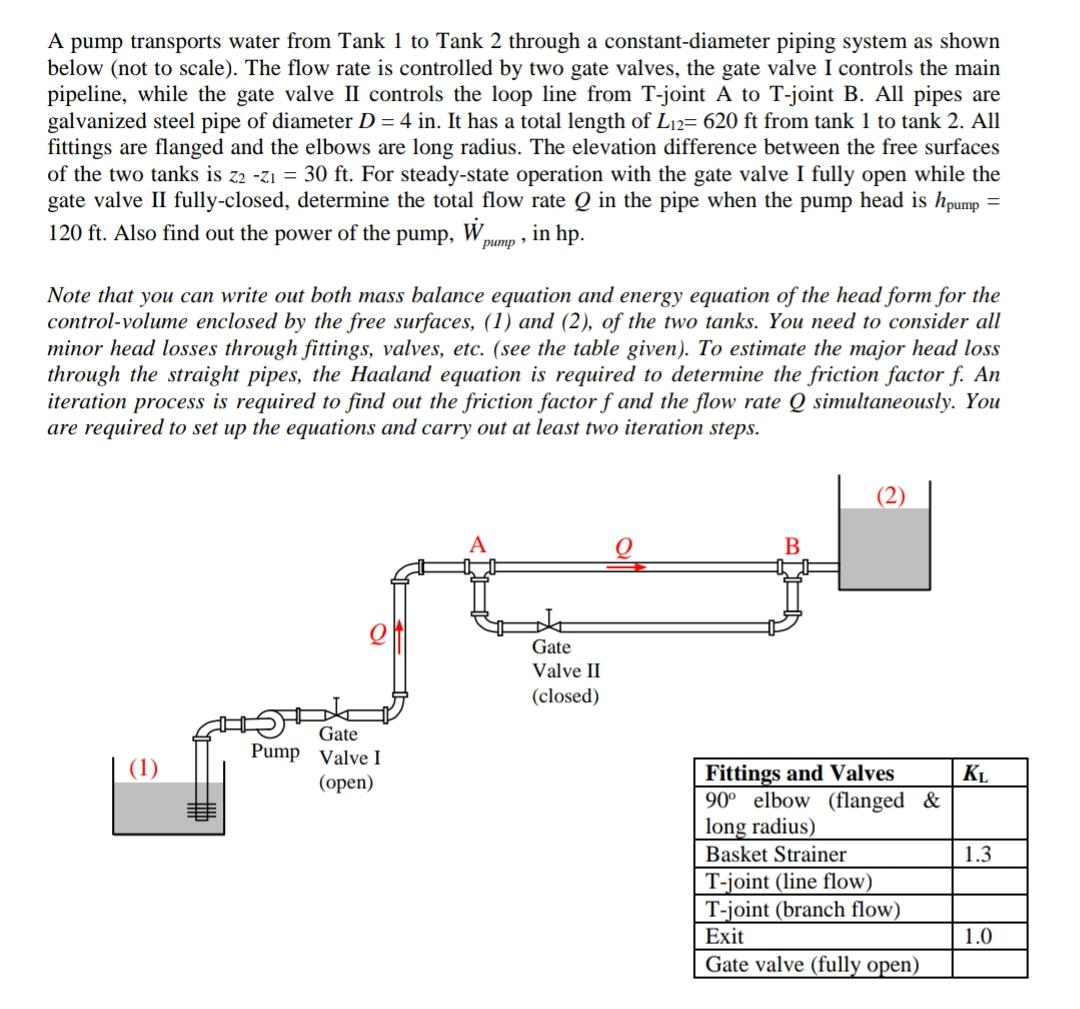A pump transports water from Tank 1 to Tank 2 through a constant-diameter piping system as shown below (not to scale). The flow rate is controlled by two gate valves, the gate valve I controls the main pipeline, while the gate valve II controls the loop line from T-joint A to T-joint B. All pipes are galvanized steel pipe of diameter D = 4 in. It has a total length of Li2= 620 ft from tank 1 to tank 2....

• Notre Dame University Faculty of Engineering Mechanical Engineering Dep Water flows through cast iron pipes and between the two tanks shown in Figure P4. The free sharp edge entrance. Bd by a dis...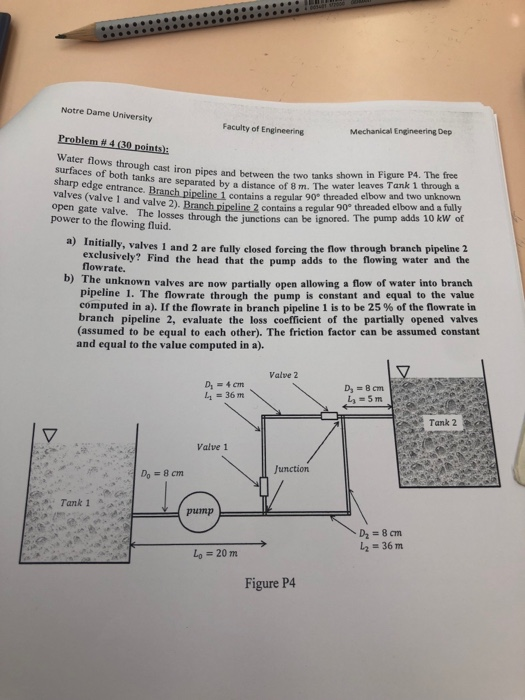Notre Dame University Faculty of Engineering Mechanical Engineering Dep Water flows through cast iron pipes and between the two tanks shown in Figure P4. The free sharp edge entrance. Bd by a distance of 8 m. The water leaves Tank 1 through a contains a regular 90 threaded elbow and two unknown a regular 90° threaded elbow and a fully Branch pipeline 1 contains a regular 90 valves (valve 1 and valve 2). Branch pipeline 2 contains open gate valve....

• Notre Dame University Faculty of Engineering Mechanical Engineering Dep Water flows through cast iron pipes and between the two tanks shown in Figure P4. The free sharp edge entrance. Bd by a dis...Notre Dame University Faculty of Engineering Mechanical Engineering Dep Water flows through cast iron pipes and between the two tanks shown in Figure P4. The free sharp edge entrance. Bd by a distance of 8 m. The water leaves Tank 1 through a contains a regular 90 threaded elbow and two unknown a regular 90° threaded elbow and a fully Branch pipeline 1 contains a regular 90 valves (valve 1 and valve 2). Branch pipeline 2 contains open gate valve....

• Notre Dame University Faculty of Engineering Mechanical Engineering Dep Water flows through cast iron pipes and between the two tanks shown in Figure P4. The free sharp edge entrance. Bd by a dis...Notre Dame University Faculty of Engineering Mechanical Engineering Dep Water flows through cast iron pipes and between the two tanks shown in Figure P4. The free sharp edge entrance. Bd by a distance of 8 m. The water leaves Tank 1 through a contains a regular 90 threaded elbow and two unknown a regular 90° threaded elbow and a fully Branch pipeline 1 contains a regular 90 valves (valve 1 and valve 2). Branch pipeline 2 contains open gate valve....

• The figure below shows a condenser/cooling tower pipe system needed to remove heat from a water...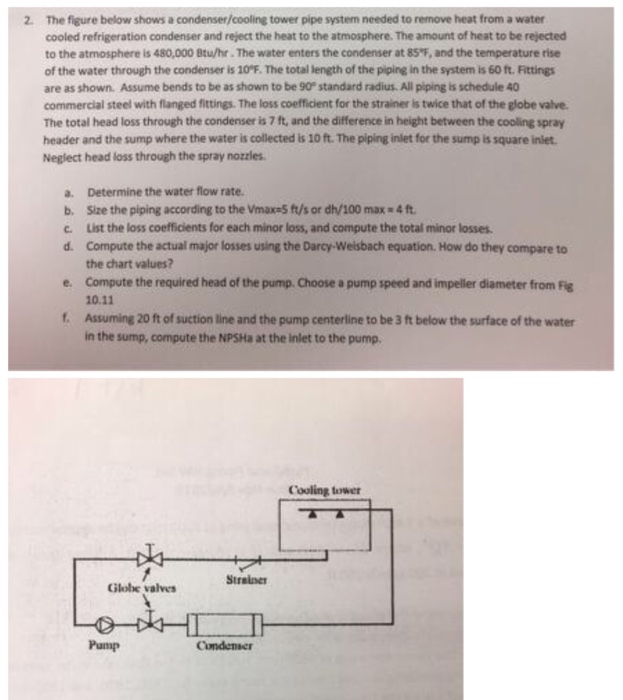The figure below shows a condenser/cooling tower pipe system needed to remove heat from a water cooled refrigeration condenser and reject the heat to the atmosphere. The amount of heat to be rejected to the atmosphere is 480,000 Btu/hr. The water enters the condenser at 85"F, and the temperature rise of the water through the condenser is 10°F. The total length of the piping in the system is 60 ft. Fittings are as shown. Assume bends to be as shown...

• Fig. Q4 shows a piping arrangement used in a food processing facility to transfer water, at 40 C, from a tank, through a heat exchanger to an elevated tank. A suitable pump is selected which, when in...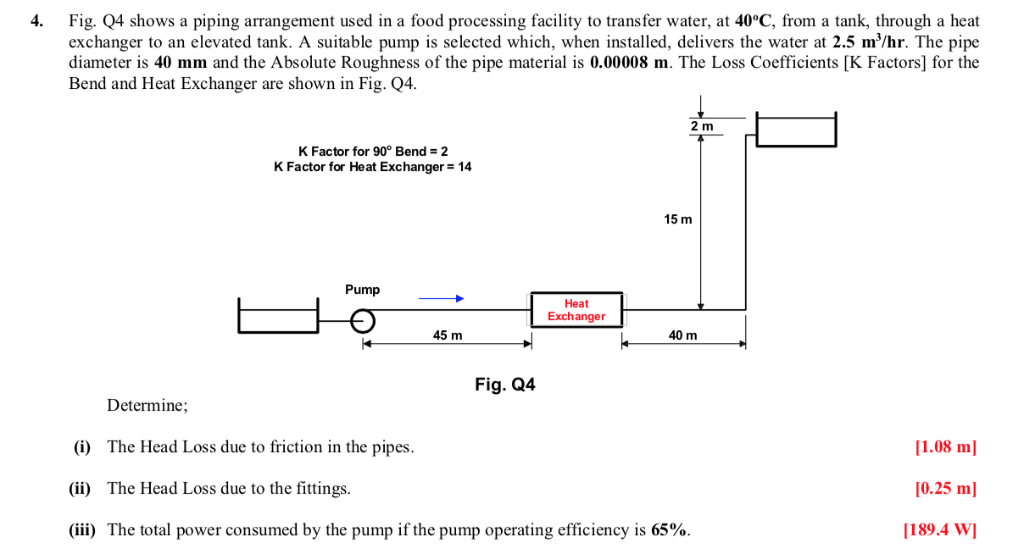Fig. Q4 shows a piping arrangement used in a food processing facility to transfer water, at 40 C, from a tank, through a heat exchanger to an elevated tank. A suitable pump is selected which, when installed, delivers the water at 2.5 m'/hr. The pipe diameter is 40 mm and the Absolute Roughness of the pipe material is 0.00008 m. The Loss Coefficients [K Factors] for the Bend and Heat Exchanger are shown in Fig. Q4 4. 2 m K...

• The system below shows a simplified schematic of an oil transfer system between tanks, from left...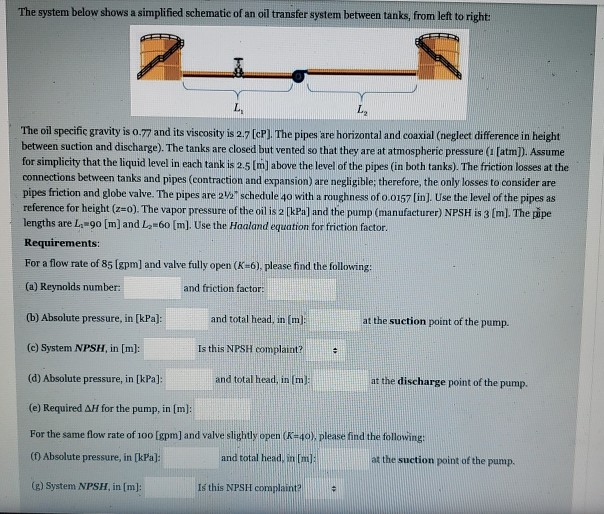The system below shows a simplified schematic of an oil transfer system between tanks, from left to right: L L The oil specific gravity is 0.77 and its viscosity is 2.7 [cP). The pipes are horizontal and coaxial (neglect difference in height between suction and discharge). The tanks are closed but vented so that they are at atmospheric pressure ([atm]). Assume for simplicity that the liquid level in each tank is 2.5 (m) above the level of the pipes (in...

• Please solve a,b,c,d,e,f 1) A water fountain is to be installed at a remote location by attaching a cast iron pipe directly to a water main through which water is flowing. The entrance to the pipe...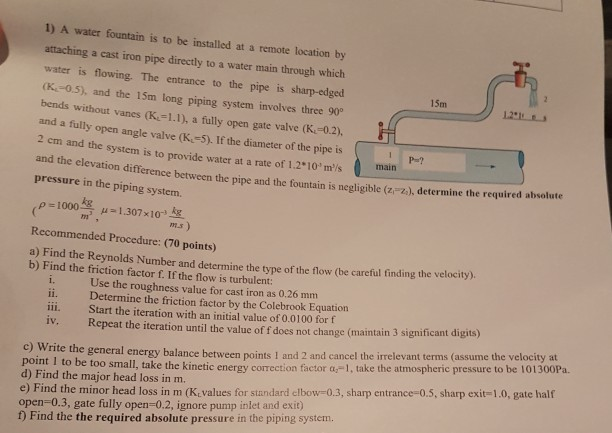Please solve a,b,c,d,e,f 1) A water fountain is to be installed at a remote location by attaching a cast iron pipe directly to a water main through which water is flowing. The entrance to the pipe is sharp-edged 15m (K-0.5), and the 15m long piping system involves three 90 bends without vanes (K1.1), a fully open gate valve (K.-0.2), and a fully open angle valve (K-5). If the diameter of the pipe is 2 em and the system is to...

• A pump that is 80% efficient pumps water from the lower tank to the upper tank...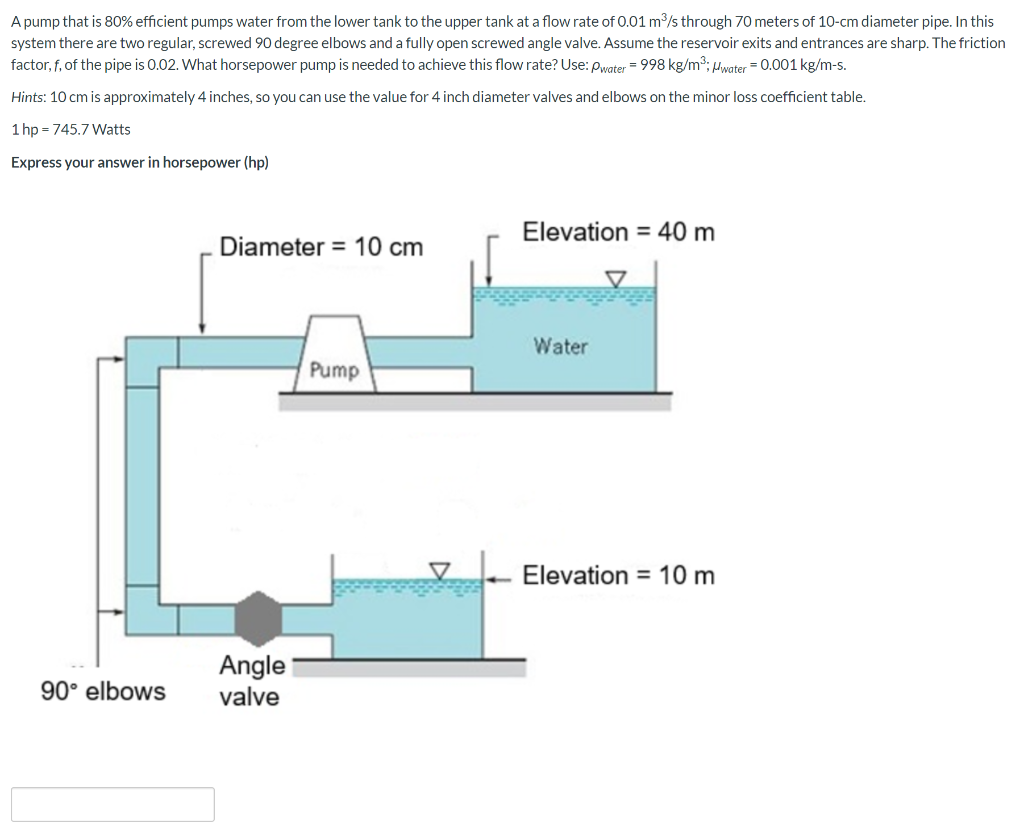A pump that is 80% efficient pumps water from the lower tank to the upper tank at a flow rate of 0.01 m/s through 70 meters of 10-cm diameter pipe. In this system there are two regular, screwed 90 degree elbows and a fully open screwed angle valve. Assume the reservoir exits and entrances are sharp. The friction factor,f, of the pipe is 0.02. What horsepower pump is needed to achieve this flow rate? Use: Pwater = 998 kg/m2; Mwater...